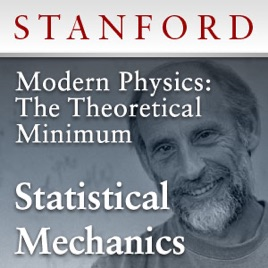10 episodes

This course is comprised of a six-quarter sequence of classes that will explore the essential theoretical foundations of modern physics. The topics covered in this course sequence will include classical mechanics, quantum mechanics, the general and special theories of relativity, electromagnetism, cosmology, and black holes. While these courses will build upon one another, each course also stands on its own, and both individually and collectively they will let students attain the “theoretical minimum” for thinking intelligently about modern physics.
Quantum theory governs the universe at its most basic level. In the first half of the 20th century physics was turned on its head by the radical discoveries of Max Planck, Albert Einstein, Niels Bohr, Werner Heisenberg, and Erwin Schroedinger. An entire new logical and mathematical foundation—quantum mechanics—eventually replaced classical physics. We will explore the quantum world, including the particle theory of light, the Heisenberg Uncertainty Principle, and the Schroedinger Equation.# Modern Physics: The Theoretical Minimum - Statistical Mechanics Stanford

• Science

This course is comprised of a six-quarter sequence of classes that will explore the essential theoretical foundations of modern physics. The topics covered in this course sequence will include classical mechanics, quantum mechanics, the general and special theories of relativity, electromagnetism, cosmology, and black holes. While these courses will build upon one another, each course also stands on its own, and both individually and collectively they will let students attain the “theoretical minimum” for thinking intelligently about modern physics.
Quantum theory governs the universe at its most basic level. In the first half of the 20th century physics was turned on its head by the radical discoveries of Max Planck, Albert Einstein, Niels Bohr, Werner Heisenberg, and Erwin Schroedinger. An entire new logical and mathematical foundation—quantum mechanics—eventually replaced classical physics. We will explore the quantum world, including the particle theory of light, the Heisenberg Uncertainty Principle, and the Schroedinger Equation.

• video
Statistical Mechanics Lecture 1 (March 30, 2009)

## Statistical Mechanics Lecture 1 (March 30, 2009)

Leonard Susskind discusses the study of statistical analysis as calculating the probability of things subject to the constraints of a conserved quantity. (March 30, 2009)

• 2 hr
• video
Statistical Mechanics Lecture 2 (April 6, 2009)

## Statistical Mechanics Lecture 2 (April 6, 2009)

Leonard Susskind overviews elementary mathematics to define a method for understanding statistical mechanics. (April 6, 2009)

• 46 min
• video
Statistical Mechanics Lecture 3 (April 13, 2009)

## Statistical Mechanics Lecture 3 (April 13, 2009)

Leonard Susskind reviews the Lagrange multiplier, explains Boltzmann distribution and Helm-Holtz free energy before oulining into the theory of fluctuations. (April 13, 2009)

• 1 hr 55 min
• video
Statistical Mechanics Lecture 4 (April 20, 2009)

## Statistical Mechanics Lecture 4 (April 20, 2009)

Leonard Susskind explains how to calculate and define pressure, explores the formulas some of applications of Helm-Holtz free energy, and discusses the importance of the partition function. (April 20, 2009)

• 1 hr 35 min
• video
Statistical Mechanics Lecture 5 (April 27, 2009)

## Statistical Mechanics Lecture 5 (April 27, 2009)

Leonard Susskind discusses the basic physics of the diatomic molecule and why you don't have to worry about its structure at low temperature. Susskind later explores a black hole thermodynamics. (April 27, 2009)

• 1 hr 47 min
• video
Statistical Mechanics Lecture 6 (May 4, 2009)

## Statistical Mechanics Lecture 6 (May 4, 2009)

Leonard Susskind explains the second law of thermodynamics, illustrates chaos, and discusses how the volume of phase space grows. (May 4, 2009)

• 1 hr 12 min

## Customer Reviews

M1shooter ,

### Great Series

Another great lecture series. I wish that there were more lectures.
The only distractor is the "off the wall" questions; which there less.